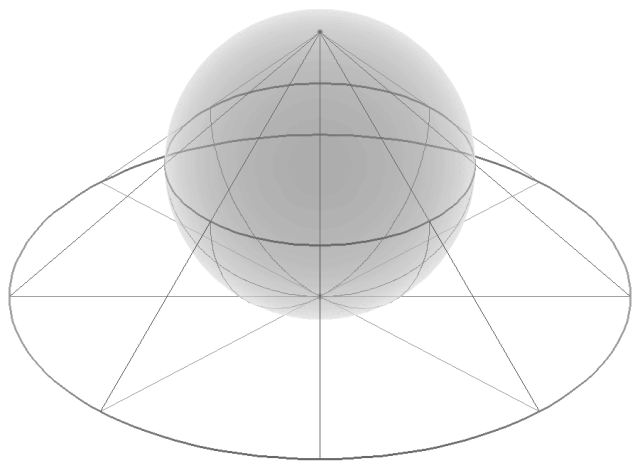Want to share your content on R-bloggers? click here if you have a blog, or here if you don't.

The Cauchy distribution (`?dcauchy` in `R`) nails a flashlight over the number lineand swings it at a constant speed from 9 o’clock down to 6 o’clock over to 3 o’clock. (Or the other direction, from 3→6→9.) Then counts how much light shone on each number.In other words we want to map evenly from `the circle (minus the top point)` onto `the line`. Two of the most basic, yet topologically distinct shapes related together.You’ve probably heard of a mapping that does something close enough to this: it’s called `tan`.Since `tan` is so familiar it’s implemented in Excel, which means you can simulate draws from a Cauchy distribution in a spreadsheet. Make a column of `=RAND()`’s (say column A) and then pipe them through `tan`. For example `B1=TAN(A1)`. You could even do `=TAN(RAND())` as your only column. That’s not quite it; you need to stretch and shift the `[0,1]` domain of `=RAND()` so it matches `[−π,+π]` like the circle. So really the long formula (if you didn’t break it into separate columns) would be `=TAN( PI() * (RAND()−.5) )`. A stretch and a shift and you’ve matched the domains up. There’s your Cauchy draw.

In R one could draw three Cauchy’s with `rcauchy(3)` or with `tan(2*(runif(3)−`.5)).What’s happening at `tan(−3π/2)` and `tan(π/2)`? The `tan` function is putting out to ±∞.

I saw this in school and didn’t know what to make of it—I don’t think I had any further interest than finishing my problem set.I saw as well the ±∞ in the output of `flip[x]= 1/x`.

• `1/−.0000...001 → −∞` whereas` 1/.0000...0001 → +∞`.

It’s not immediately clear in the `flip[x]` example but in `tan[x/2]` what’s definitely going on is that the angle is circling around the top of the circle (the hole in the top) and the flashlight of the Cauchy distribution could be pointing to the right or to the left at a parallel above the line.

Why not just call this ±∞ the same thing? “Projective infinity”, or, the hole in the top of the circle.